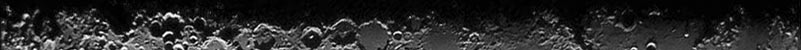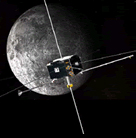The Mission# Instruments

Digital Fields Board (component of EFI, SCM)

Q: What are the frequency bands for the Filter bank (FBK) data?
A: For SCM and EDC data, the relevant frequencies (-6 dB) are:

Band fmin (Hz) fcenter(Hz) fmax (Hz) Bandwidth (Hz)

1 1390 2689 5994 4604
2 316 572 904 587
3 80.2 144.2 227.4 147.2
4 20.1 36.2 57.0 36.9
5 5.04 9.05 14.3 9.23
6 1.26 2.26 3.57 2.31

For EAC data, the -6 dB frequencies are similar, except for the maximum frequency and bandwidth of the top band:

Band fmin (Hz) fcenter (Hz) fmax (Hz) Bandwidth (Hz)

1 1262 2298 3672 2410
2 318 574 906 586
3 80.4 144.6 227.8 147.4
4 20.2 36.2 57.0 36.8
5 5.04 9.06 14.3 9.22
6 1.26 2.26 3.57 2.31

Q: What are the frequency bands for the Fourier power spectra (FFT)?
A: The FFT frequency bands depend on the mode in which the DFB has been commanded to operate.  There can be 16, 32 or 64 bands, spaced pseudo-logarithmically over the range 0-4096 Hz for the SCM and EDC data, and over the range 0-8192 Hz for the EAC data.  There are tables with the exact frequencies in the Space Science Reviews article by Cully et al .

Q: What is the difference between EDC and EAC data?
A: The EDC data is sampled at 8192 samples per second, and has a maximum range of roughly 400 mV/m for the radial booms at low frequency (below a few hundred Hz) and roughly 800 mV/m at high frequency.  The EAC data is sampled at 16384 samples per second, and has a sensitivity 6 times that of the EDC data.  The EAC data has a high-pass filter at 10 Hz, and so should not be used below this frequency.

Q: Why are the units for the FBK data |mV/m| or |nT|, while the units for the FFT data are (mV/m)2 or nT2?
A: The FFT data are true wave powers calculated using a (fast) Fourier transform, and are therefore in power units.  The FBK data are calculated as the mean of the absolute value of the bandpass-filtered signal, and are therefore in units of amplitude. To convert the FBK data to wave amplitudes, multiply by pi/2.  To convert to mean-square power, square the FBK data and multiply by pi2/8.

Q: What is the accuracy for the FFT and FBK data?
A: The FBK and FFT data are fully corrected for the known frequency-dependent instrument responses.  The AC response of the SCM instrument (and hence the accuracy of the SCM FBK and FFT data) is known from both ground and in-flight calibrations, and the amplitudes are accurate to within a few percent.  The AC response of the EFI instrument cannot be fully tested on the ground, since the required test chamber would be prohibitively large. Hence, the EFI AC response is calculated based on known properties of the instrument (geometry, input capacitance, etc.), rather than being measured directly. This response function has been verified in-flight by cross-calibrating with the SCM data during intervals of well-identified plasma waves with known E/B ratios. At frequencies above 500 Hz or below 100 Hz, the EFI amplitudes are accurate to within a factor of 2.  From 100 to 500 Hz, the response function changes rapidly and the EFI amplitudes are consequently only accurate to within a factor of 4 in this region.

Q: What are the HF quantities in the FBK data?
A: The HF quantities are measurements of the electric wave pseudo-power (mean amplitude) in the band from 30 kHz to 500 kHz. Two quantities are reported in the telemetry: the peak value and the average value of the amplitude in this band during the sampling interval.

Q: What is the smallest (or largest) signal that can be seen in the FBK and FFT data?
A: For both the FBK and FFT data, the maximum range is the full EFI or SCM instrument range.  For the FBK data, the uncompressed dynamic range is 19 bits.  Hence, the smallest amplitude that can be seen is 1/8 of an ADC unit. For the FFT data, the uncompressed powers are 36 bits wide, so the smallest amplitude is 1/16 of an ADC unit.  The relation between ADC units and physical quantities is frequency-dependent, but at low frequencies, 1 ADC corresponds to roughly 0.01 mV/m for EDC (radial booms), 0.002 mV/m for EAC (radial booms) and 0.2 pT for SCM.

Q: Where can I find more information on the FBK and FFT data?
A: The FBK and FFT data come from the EFI and SCM instruments. More information on these instruments can be found in the instrument descriptions on this site and in the Space Science Reviews articles by Bonnell et al  and Le Contel et al . The data processing is performed by the Digital Fields Board, and the Space Science Reviews article by Cully et al  covers much of the information specific to the FBK and FFT data.